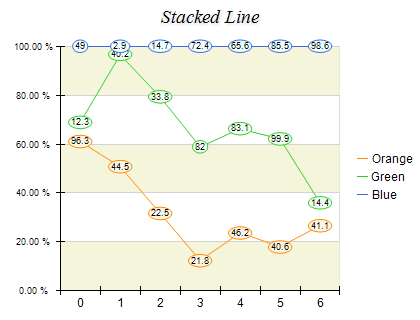﻿ Stacked Percent Line
Nevron .NET Vision
Chart for .NET / User's Guide / Chart Types / Line / Stacked Percent Line

In This Topic
Stacked Percent Line
In This Topic

Stacked Percent Line charts represent the relative contribution of data series to the total for each category. The series are displayed as sequences of straight line segments. Each line series is stacked on top of the previous one. The height of the uppermost line is always the full height of the chart. The Y scale range is always from 0 to 1. The following figure displays a Stacked Percent Line chart:Figure 1.

Creating the stacked percent line chart

Stacked Percent Line charts are displayed with several NLineSeries objects. The MultiLineMode property of the first line series must be set to MultiLineMode.Series. The MultiLineMode property of the subsequent line series must be set to MultiLineMode.StackedPercent. The following example demonstrates how to create a stacked percent line chart with three stacks:

C#
Copy Code
```// obtain a reference to the Cartesian chart that is created by default
NCartesianChart chart = (NCartesianChart)chartControl.Charts;

line1.MultiLineMode = MultiLineMode.Series;
line2.MultiLineMode = MultiLineMode.StackedPercent;
line3.MultiLineMode = MultiLineMode.StackedPercent;
```
Visual Basic
Copy Code
```' obtain a reference to the Cartesian chart that is created by default
Dim chart As NCartesianChart = chartControl.Charts(0)

Dim line1 As NLineSeries = chart.Series.Add(SeriesType.Line)
Dim line2 As NLineSeries = chart.Series.Add(SeriesType.Line)
Dim line3 As NLineSeries = chart.Series.Add(SeriesType.Line)

line1.MultiLineMode = MultiLineMode.Series
line2.MultiLineMode = MultiLineMode.StackedPercent
line3.MultiLineMode = MultiLineMode.StackedPercent
```
Stacked Percent Scaling

When a line series is displayed in stacked percent mode the min value of the series is always 0 and max value of the series is always 1. In order to display the texts on the PrimaryY axis as percents you have to use the following code:

C#
Copy Code
```NStandardScaleConfigurator sc = (NStandardScaleConfigurator)chart.Axis(StandardAxis.PrimaryY).ScaleConfigurator;
sc.LabelValueFormatter = new NNumericValueFormatter(NumericValueFormat.Percentage);
```
Visual Basic
Copy Code
```Dim sc As NStandardScaleConfigurator = chart.Axis(StandardAxis.PrimaryY).ScaleConfigurator
sc.LabelValueFormatter = New NNumericValueFormatter(NumericValueFormat.Percentage)
```
Stacks with negative values
Stacked percent line charts do not support negative values, which means that all values are internally converted to their absolute value.
Formatting Commands

The following formatting commands inherited from the NSeries class have different meaning when a line series is stacked:

<total> - displays the absolute sum of the values in the current stack.
<cumulative> - displays the sum of the values up to the current stack value.
<percent> - displays the percent contribution of the value to the total pile sum.

Related Examples
Windows forms: Chart Gallery\Line\Stacked Line